# happy/sad: An Illustrative DICE Example

## Background

The model describes two diseases: sadness and flu. In the model, the mood influences the probability to get the flu. On the other hand, having the flu increases the probability to die. The following schematics shows the model events and their mutual interactions.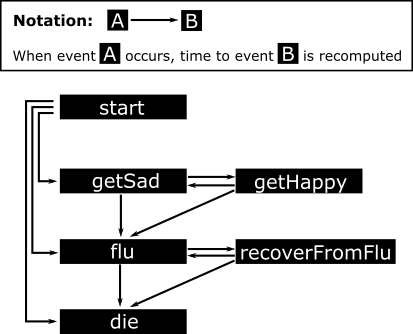## The mechanism of the model

An individual is described by several conditions: age, sex, status (health status, healthy or flu), mood (happy or sad) and treatment (no or beNICE). These conditions change over time due to the occurence of events.

At the beginning, event times for getSad, flu and die are determined from random distributions. When getSad occurs, the subject’s mood changes from happy to sad and treatment with beNICE begins. Event times for flu and getHappy are recomputed. Accordingly, when the person gets happy, treatment stops, events flu and getSad are rescheduled.

When the person gets the flu, the health status changes to flu and the counter nflu for the number of how often the patient has been infected by the flu is increased by one. The event time for die is recomputed with the new health status and the event time for recoverFromflu is drawn. Accordingly, when the person recovers from the flu, it’s health status switches back to healthy and the events flu and die are rescheduled.

Between all events, age, utility and cost are accumulated.

# Model set-up

For modeling with the RHEM package we need to load it with library(). The dplyr package is also useful.

library(RHEM)
library(dplyr)

In the model we are going to need the following look-up tables:

data("LifeExpectancy")

treatment = c("no", "beNICE"),
value = c(48, 12)
)

Cost <- data.frame(
treatment = c("no", "beNICE"),
status = c("healthy", "healthy", "flu", "flu"),
value = c(0, 10, 5, 15)
)

Note: Look-up tables will in future be part of the model definition, similar to Function()s.

## Model-building functions

A DICE model in the RHEM framework is set up step by step. Several elements like Conditions(), Parameters(), Event(), Rule() and Observation() are put together by the + operator.

### Conditions

Conditions desribe properties of a simulated subject or patient that characterize the subject and change over time. For better readability, conditions can be assigned to different categories using the name argument. For the example, the conditions look as follows:

dice <-

Conditions(
"Patient",
age           = Continuous(50, unit = "years"),
sex           = Categorical("male"   , levels = c("male", "female")),
status        = Categorical("healthy", levels = c("healthy", "flu")),
mood          = Categorical("happy"  , levels = c("happy", "sad")),
treatment     = Categorical("no"     , levels = c("no", "beNICE"))
) +

Conditions(
"Disease",
timeToflu     = Continuous(48, unit = "months"),
nflu          = Continuous(0 , unit = "")
) +

Conditions(
"Health_Economic",
cost          = Continuous(0 , unit = "$"), utility = Continuous(0 , unit = "") )  The first argument of Conditions() defines the category name. The following arguments have the structure condition_name = value. The condition_name can be used in the later definition of events. The value is used to initialize the conditions. Values can either be Continuous or Categorical. The above dice can already be simulated and plotted albeit yielding a trivial result: dice %>% Simulate(0:10) %>% Compare(names(dice$conditions$Patient)) %>% Plot()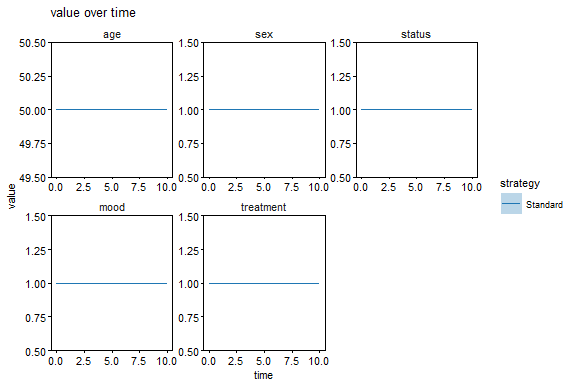Note: During modeling it is helpful to run the above line every time a new event is added. This way, it is possible to control if the event definition causes the expected behavior. ### Parameters Parameters describe values that are constant over time. Same as for conditions, parameters can be attributed to different categories. In our example the parameters read: dice <- dice + Parameters( "Disease", timeTosad = Continuous(30, unit = "months") ) + Parameters( "Standard_of_Care", mytreatment = Categorical("no", levels = c("no", "beNICE")), strategy = "SoC" ) + Parameters( "new treatment", mytreatment = Categorical("beNICE", levels = c("no", "beNICE")), strategy = "beNICE" ) The structure of the Parameters() function is identical to Conditions(). Values can either be explicitely defined as Continuous() or as raw numbers. Here, we introduce two strategies (SoC and beNICE). The parameter mytreatment is specified per strategy. Note: The handling of strategies will in the future be simplified. ### Functions The user can define functions which are callable from inside events. Usually, these functions serve the purpose to outsource lengthy computations which have to be repeated several times within different events. dice <- dice + Function("dt", # The increase in years per unit simulation time function(t) 1/12) + Function("QoL", # Quality of Life assessment function(myage, mysex, mystatus, mymood) { age0 <- c(male = 70, female = 80) lambda <- c(male = 15, female = 20) factor1 <- c("healthy" = 1, "flu" = .1) factor2 <- c("happy" = 1, "sad" = .1) factor1[mystatus]*factor2[mymood]/(exp((myage - age0[mysex])/lambda[mysex]) + 1) }) Here, the function dt is defined as that function that returns the time step in years per simulation time step which are months. The function QoL computes quality of life as a function of the current age, sex, health status and mood. ### Events Events make the heart of the model. Ideally, event statements can be read from top to bottom explaining the model mechanism. It is usefule to have inline documentation to provide people with information about the processes. dice <- dice + Event( "start", note = "Initliaze the DICE simulation determining time points of the scheduled events.", getSad = Lifetime(scale = timeTosad), die = 12*Lifetime(scale = Evaluate("LifeExpectancy", age, sex, status)), flu = Lifetime(scale = timeToflu) ) + Event( "getSad", note = "Subject gets sad. The mood changes. The expected time to flu decreases. treatment beNICE starts. Events getHappy and flu get re-scheduled.", mood = "sad", timeToflu = 12, treatment = mytreatment, getHappy = Lifetime(scale = Evaluate("sadTohappy", treatment)), flu = Lifetime(scale = timeToflu) ) + Event( "getHappy", note = "Subject gets happy. The mood changes. The expected time to flu is set back. treatment stops. Events getSad and flu get re-scheduled.", mood = "happy", timeToflu = 24, treatment = "no", getSad = Lifetime(scale = timeTosad), flu = Lifetime(scale = timeToflu) ) + Event( "flu", note = "Subject gets the flu. Time point of recovery is taken from random distribution according to expected duration. The event die is rescheduled with the increased risk when infected by the flu.", status = "flu", nflu = . + 1, RecoverFromflu = Lifetime(scale = 1), die = Lifetime(scale = 12*Evaluate("LifeExpectancy", age, sex, status)) ) + Event( "RecoverFromflu", note = "Subject recovers from flu. The next flu is scheduled. The event die is re-scheduled according to the lower risk to die when being healthy.", status = "healthy", flu = Lifetime(scale = timeToflu), die = Lifetime(scale = 12*Evaluate("LifeExpectancy", age, sex, status)) ) + Event( "die", note = "The subject dies. The simulation stops right after the event.", stop = 0 ) ### Rules Rules is a special event that is executed after each event. Rules are useful to update health and economic values or the subject’s age. The structure of the Rule() function is identical to Event(). dice <- dice + Rule( "General", note = "Age, utility and cost are accumulated over time since the last event.", age = . + Accumulate("dt", time), utility = . + Accumulate("QoL", age, sex, status, mood)/12, cost = . + Accumulate("Cost", treatment, status)/12 ) ### Observations Observations are expressions that can be evaluated on top of the simulation result. Observations can change over time. In this sense, they are treated as conditions. However, as opposed to conditions, they do not impact the evolution of events but can be deduced from the time-series of conditions after the simulation. dice <- dice + Observation( "Health_Economic", note = "Compute utiliy per year and cost per year by differentiating the total utility and cost.", utility_per_year = Differentiate(utility, time), cost_per_year = Differentiate(cost, time) ) ## The Model() function The RHEM framework provides a Model() function to set up both DICE and Markov models. The Model() function has mandatory arguments name, author, date and note to define a short name of the model, state author and date of creation and provide a detailed description of the model’s purpose. Finally, the model itself is defined within the dots argument using combining model-building functions. In this vignette, the model construction was already done before, yielding the object dice. Usually, the concatenation of conditions, parameters, events, rules and observations can be done within the dots argument. model <- Model( "happysad", author = "Daniel Kaschek", date = "3 May 2018", note = "The DICE model describes two states of mood, 'happy' and 'sad' and two health states 'healthy' and 'flu'. The model is implemented as a time-to-event model, where “getHappy” and “getSad” occur randomly as is getting the flu and recovering from flu. There are two strategies, 'SoC' (standard of care) and 'beNICE' (new treatment).", dice ) # Analysis set-up Each analysis follows the same structure: Simulate(), Compare() and Plot() are concatenated by the pipe operator from the dplyr package. The purpose of the individual analysis is further specified by the arguments of the Simulate() and Compare() function. ## Single-trace analysis The first analysis is supposed to produce two individual traces of conditions over time, one for each strategy. The patient conditions names(model$conditions$Patient) are compared by their values. times <- 0:60 simulation <- model %>% Simulate(times, n = 1) simulation %>% Compare(names(model$conditions\$Patient), by = "value") %>%
Plot()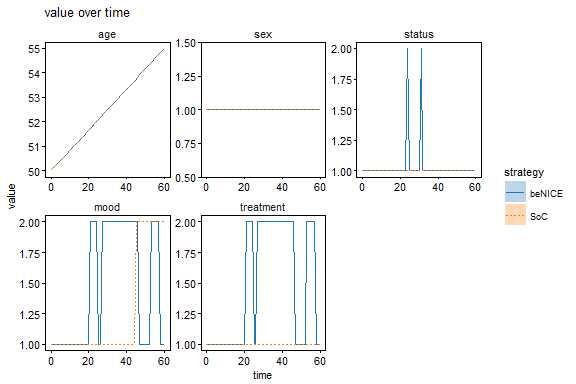## Multi-trace analysis

The second analysis runs the same simulation n = 100 times. First, the Compare() function is used to compare nflu (how often the person was in total affected by the flu), cost and utility. The reported value for the 50 individuals is the mean with quantiles according to a coverage of 68%.

times <- 0:60
simulation <- model %>% Simulate(times, n = 10)
simulation %>%
Compare(c("nflu", "cost", "utility"), by = "value", value = "mean", sigma.value = "68%") %>%
Plot()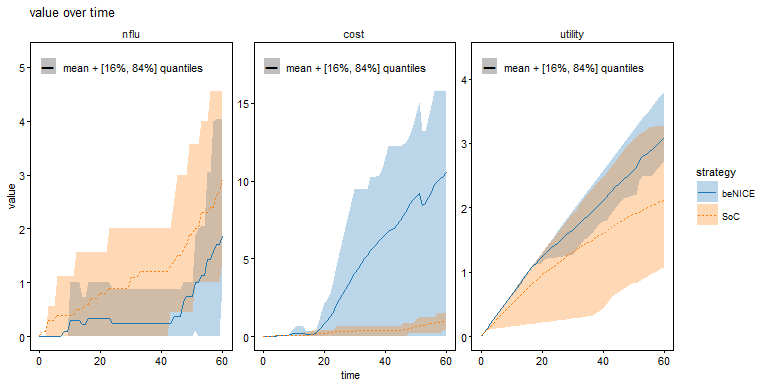The beNICE strategy causes higher costs but has also a higher utility. The number of infections by the flu within five years is slightly decreased.

Second, the condition mood is compared. The reported value is the proportion of individuals being in either level ov mood (1 = happy, 2 = sad).

simulation %>%
Compare("mood", by = "value", value = "proportion", match.tol = 2) %>%
Plot()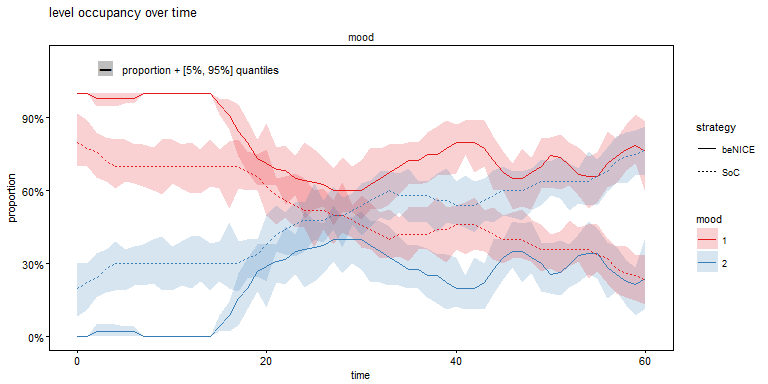With the new treatment, individuals are found to be happier than without the treatment